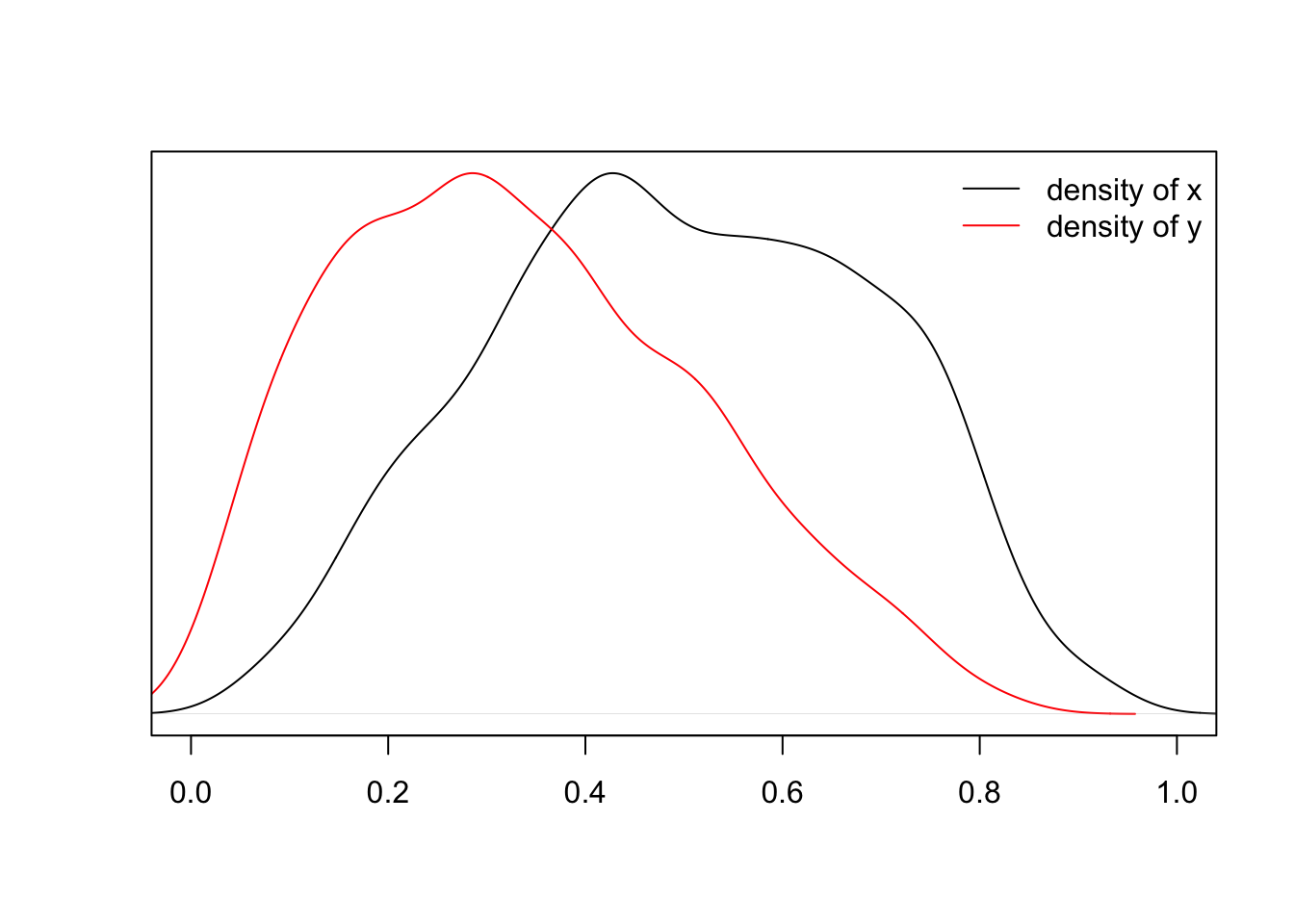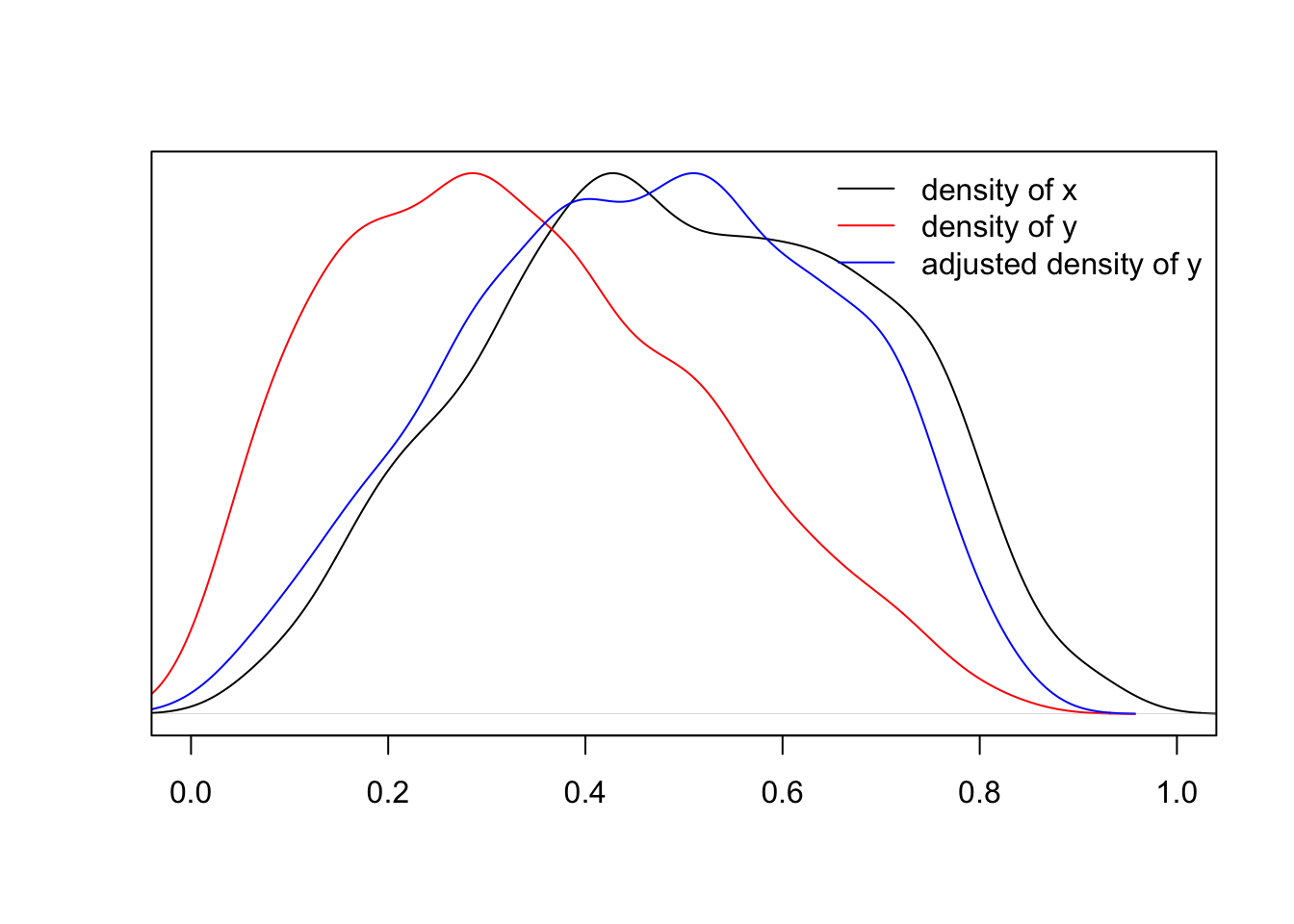## 1. Introduction

In validation studies, performance indices are usually estimated by simply applying a model to a validation set; however, if the distribution of risk factors (or predictors) in the validation set is different from that on the relevant clinical population, such an approach may not provide a useful estimate of the predictive performance. For example, we sometimes observe unexpectedly poor performance in a validation set because of 1) overfitting in the development set (i.e., optimistic bias), 2) difference in distribution of the predictors between the development and validation sets, and 3) sampling variability. Unfortunately, the current analytical practice does not take the second issue into account and thus sometimes results in the inappropriate rejection of a sound and potentially useful prediction model

This document illustrates how to estimate prediction performance metrics, adjusting for differnece in distribution of the predicitors package among datasets and how to implement the method with our APPEsimation package. The package currently consits of two main functions: appe.lm and appe.glm. The function appe.lm calculates \(L_1\) and \(L_2\) errors for models with a continuous outcome, and the function appe.glm is for a binary outcome and calculates \(C\)-index. Note that the prediction models need to be build by using using lm funtion for a continous outcome and glm function for a binary outcome to use appe.lm and appe.glm, respectively.

## 2. How to install package

For implementation in R, APPEstimation package and densratio package are required. These can be installed from the CRAN as follows.

``````install.packages("APPEstimation")
install.packages("densratio")``````

## 3. Illustrating examples

We illustrate the method with the following simple numerical example. First, two sets of data, \(x\) and \(y\), are sampled from beta distributions with different parameters. \(x\) is sampled from Beta(3, 3), and \(y\) is sampled from Beta(2, 4). The estimated densities are plotted on the figure below. Distribution of \(x\) is symmetric while that of \(y\) is skewed to the left.

``````set.seed(10)

x  = rbeta(1000, 3, 3)
y  = rbeta(1000, 2, 4)
xd  = density(x)
yd  = density(y)

plot(xd, xlim=c(0,1), ylim=range(xd\$y), col=1, axes=FALSE, xlab='', ylab='', main='')
par(new=TRUE)
plot(yd, xlim=c(0,1), ylim=range(yd\$y), col=2, axes=FALSE, xlab='', ylab='', main='')
axis(1)
box()
legend("topright", c("density of x", "density of y"), col=c(1, 2), lwd=1, bty="n")``````Density of \(x\) and \(y\) is clearly different. By applying density ratio method, density of \(y\) is adjusted to that of \(x\).

The density ratio estimation can be conducted by the following code.

``````dr  = densratio(x, y, verbose=FALSE)
wgt = dr\$compute_density_ratio(y)``````

Here, `wgt` is the density ratio estimates, which can be used as weights to adjust the distribution of \(y\) to that of \(x\). The adjusted density of \(y\) is shown in the follwoing figure (blue solid line), which appears to be similar to the density of \(x\) (black solid line).

``````ydw = density(y, weights=wgt/sum(wgt))

plot(xd, xlim=c(0,1), ylim=range(xd\$y), col=1, axes=FALSE, xlab='', ylab='', main='')
par(new=TRUE)
plot(yd, xlim=c(0,1), ylim=range(yd\$y), col=2, axes=FALSE, xlab='', ylab='', main='')
par(new=TRUE)
plot(ydw, xlim=c(0,1), ylim=range(ydw\$y), col=4, axes=FALSE, xlab='', ylab='', main='')
axis(1)
box()
legend("topright", c("density of x", "density of y", "adjusted density of y"), col=c(1, 2, 4), lwd=1, bty="n")``````Thus, by using the density ratio as the weights, we can calculate how to perform the prediction model when the distribution of the predictor is close to \(x\) using a new data that is indepedent of \(x\) and possibly the distribtion of the new data is different from the distribution of \(x.\)

### 3.1 \(L_1\) and \(L_2\) errors

First example is to estimate adjusted \(L_1\) and \(L_2\) errors for a linear model with continuous outcome. Below is the sample code.

``````library(densratio)
library(APPEstimation)

set.seed(100)

# generating development data
n0  = 100
Z   = cbind(rbeta(n0, 3, 3), rbeta(n0, 3, 3))
Y   = apply(Z, 1, function(xx) { rlnorm(1, sum(c(1, 1) * xx), 0.3) })
dat = data.frame(Za=Z[,1], Zb=Z[,2], Y=Y)

# the model to be evaluated
mdl = lm(Y~ Za + Zb, data=dat)

# generating validation dataset
n1   = 100
Z1   = cbind(rbeta(n0, 3.5, 2.5), rbeta(n0, 3.5, 2.5))
Y1   = apply(Z1, 1, function(xx) { rlnorm(1, sum(c(1, 1) * xx), 0.3) })
dat1 = data.frame(Za=Z1[,1], Zb=Z1[,2], Y=Y1)

# calculation of L1 and L2 for this model
appe.lm(mdl, dat, dat1, reps=0)``````

The results from appe.lm are returned in matrix form as follows.

``````##                           Estimate
## L1                           0.853
## L1 adjusted by score         0.724
## L1 adjusted by predictors    0.791
## L2                           1.386
## L2 adjusted by score         1.021
## L2 adjusted by predictors    1.213``````

The \(L_1\) and \(L_2\) errors calcualted with development data were 0.695 and 0.838, respectively.

“L1” and “L2” indicate non-adjusted versions of \(L_1\) and \(L_2\) erros calcurated with the external validaton dataset `dat1`, which were 0.853 and 1.386, respectively. “L1 adjusted by score” and “L2 adjusted by score” indicate adjusted versions by linear predictors distribution, and “L1 adjusted by predictors” and “L2 adjusted by predictors” indicate adjusted versions by predictor distributions (multi-dimensionally). Comparing these results, crude estimates are fairly high with respect to the adjusted estimates.

To obtain confidence intervals for these metrics, please set positive integer values to the argument `reps`. “Percentile” indicates confidence intervals calculated by the percentile method, and “Approx” indicates approximated versions by Normal distribution.

### 3.2 \(C\)-statistics

Second example is \(C\)-statistics calculation for a generalized linear model with binary outcome.
Again, below is the sample code.

``````library(densratio)
library(APPEstimation)

set.seed(100)

# generating learning data
n0  = 100
Z   = cbind(rbeta(n0, 5, 5), rbeta(n0, 5, 5))
Y   = apply(Z, 1, function (xx) { rbinom(1, 1, (1/(1+exp(-(sum(c(-2, 2, 2) * c(1, xx) )))))) })
dat = data.frame(Y=Y, Za=Z[,1], Zb=Z[,2])

# the model to be evaluated
mdl = glm(Y~., binomial, data=dat)

# validation dataset, with different centers on predictors
n1   = 100
Z1   = cbind(rbeta(n1, 6, 4), rbeta(n1, 6, 4))
Y1   = apply(Z1, 1, function (xx) { rbinom(1, 1, (1/(1+exp(-(sum(c(-2, 2, 2) * c(1, xx) )))))) })
dat1 = data.frame(Y=Y1, Za=Z1[,1], Zb=Z1[,2])

# calculation of L1 and L2 for this model
appe.glm(mdl, dat, dat1, reps=0)``````

The results from appe.glm are returned in matrix form as follows.

``````##                            Est
## Cstat                    0.735
## C adjusted by score      0.674
## C adjusted by predictors 0.662``````

Non-adjusted estimates of \(C\)-statistics with the validation data is 0.735 while it is 0.679 with the development data. Because we know the true model is the same in both development and validation, this difference is due to the difference in distribution ofpredictors.

Confidence interval calculations is the same as written in \(L_1\) error section.

The following figure illustrates the adjustment of the distribution of the risk score.

``````lp  = predict(mdl)
lp1 = predict(mdl, newdata=dat1)
wgt = densratio(lp, lp1, verbose=FALSE)\$compute_density_ratio(lp1)

lpd  = density(lp)
lp1d = density(lp1)
lp1w = density(lp1, weights=wgt/sum(wgt))

xl = range(c(lpd\$x, lp1d\$x, lp1w\$x))
yl = range(c(lpd\$y, lp1d\$y, lp1w\$y))

plot(lpd, xlim=xl, ylim=yl, col=1, axes=FALSE, xlab='', ylab='', main='')
par(new=TRUE)
plot(lp1d, xlim=xl, ylim=yl, col=2, axes=FALSE, xlab='', ylab='', main='')
par(new=TRUE)
plot(lp1w, xlim=xl, ylim=yl, col=2, lty=2, axes=FALSE, xlab='', ylab='', main='')
axis(1)
box()
legend("topright", c("learning data", "testing data", "testing data (adjusted)"), col=c(1, 2, 2), lty=c(1, 1, 2), bty="n")``````